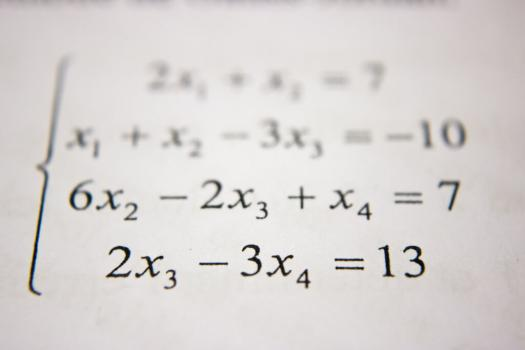# Take Our Quiz About The Theory Of Hopf Algebra

10 Questions | Total Attempts: 106SettingsThe theory of Hopf Algebra is just another extension of the algebra you already know about. It is actually described as a representation of the underlying associate algebra of abstract algebra. This might sound like something complicated for those who are not really into math, but what about you? What do you know about the theory of Hopf Algebra? Try our quiz and see if you are updated about it.

• 1.
What's abstract algebra?
• A.

It's the study of problems.

• B.

It's the study of curves.

• C.

It's the study of algebraic structures.

• D.

It's the study of distances.

• 2.
What is associate algebra?
• A.

It's an algebraic structure with compatible operation of addition, multiplication, and a scaler multiplication by elements in some fields.

• B.

It's an algebraic structure with incompatible operation of addition, multiplication, and a scaler by elements in some fields.

• C.

It's an algebraic structure with compatible operation of addition, multiplication, by elements in some fields.

• D.

It's an algebraic structure with compatible operation by elements in some fields.

• 3.
What's a vector space?
• A.

It's a collection of objects called vectors, which may be added together and multiplied by numbers called scalars.

• B.

It's a collection of vectors, which may be added together and multiplied by numbers called scalars.

• C.

It's a collection of vectors, which may be added together and multiplied by numbers.

• D.

It's a collection of objects, which may be multiplied by numbers called scalars.

• 4.
What's a function?
• A.

It's a moment between categories.

• B.

It's a map between categories.

• C.

It's a gap between categories.

• D.

It's a table between categories.

• 5.
What's the other term for coalgebras?
• A.

Alphas

• B.

Omegas

• C.

Cogebras

• D.

Vectors

• 6.
What's the other term for Einstein notation?
• A.

Einstein sum.

• B.

Einstein summation.

• C.

Einstein convention.

• D.

Einstein summation convention.

• 7.
What's a monodoil category?
• A.

It's a category C equipped with a vector.

• B.

It's a category C equipped with a factor.

• C.

It's a category C equipped with a bifunctor.

• D.

It's a category C equipped with a trifunctor.

• 8.
What's a homomorphism?
• A.

It's between 2 associative algebras, A and B over a field K.

• B.

It's between 3 associative algebras, A and B over a field K.

• C.

It's between 4 associative algebras, A and B over a field K.

• D.

It's between 2 associative algebras, A and B over a field C.

• 9.
What's bialgebra?
• A.

It's a space over K which is both a unital associative

• B.

It's a vector space over K.

• C.

It's a vector space over M which is both a unital associative

• D.

It's a vector space over K which is a unital associative

• 10.
What's a dual representation?
• A.

It's defined over the dual vector space C*

• B.

It's defined over the dual vector space V*

• C.

It's defined over the dual vector space P*

• D.

It's defined over the triple vector space V*

Related TopicsBack to top